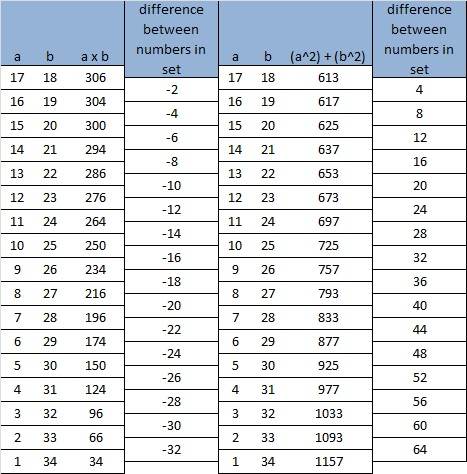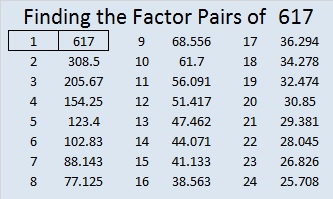# 617 Do You See a Pattern?

If you divide any even square number by 4, there will be no remainder.

If you divide any odd square number by 4, the remainder is always 1.

So if you add an even square number to an odd square number and divide the sum by 4, the remainder will ALWAYS be 1.

If you get a remainder of 1 when you divide a prime number by 4, it will ALWAYS be the sum of two squares. If you get a remainder of 1 when you divide a composite number by 4, it MIGHT be the sum of two squares.  (Note: If the last 2 digits of a number is divisible by 4, then the entire number is divisible by 4. The same thing can be said about remainders when dividing by 4.)

The last two digits of 617 yield a remainder of 1 when they are divided by 4, so 617 is a candidate for being the sum of two squares. To find out if it is, I wrote out all the square numbers less than 617:

1, 4, 9, 16, 25, 36, 49, 64, 81, 100, 121, 144, 169, 196, 225, 256, 289, 324, 361, 400, 441, 484, 529, and 576.

Then I calculated 617 – 1 – 3 – 5 – 7 – 9 – 11 – 13 – 15 – 17 – 19 – 21 – 23 – 25 – 27 – 29 – 31, and stopped there because 361 or 19^2 appeared on the calculator screen.

Since (31 + 1)/2 = 16, I knew that 19² + 16² = 617.

Having recently seen that 18² + 17² = 613, I was surprised. The difference between 18 and 17 is one, the difference between 19 and 16 is three, and the difference between 617 and 613 is four. I immediately thought of some multiplication facts:

• 7 x 8 = 56
• 6 x 9 = 54     (Note: 56 – 54 = 2)
• 5 x 10 = 50   (54 – 50 = 4)
• 4 x 11 = 44    (50 – 44 = 6)
• 3 x 12 = 36    (44 – 36 = 8)

In the standard multiplication table these facts can be seen on a single diagonal. The facts above have been highlighted in red. (The table is symmetrical so the diagonal actually extends in both directions.)If we look at the orange diagonal on the multiplication table above, we see 42, 40, 36, 30, 22, and 12. The differences between each consecutive member in that set are 2, 4, 6, 8, and 10. No matter which diagonal we choose the differences in the numbers follow that same pattern.

I asked myself, “Would there be a similar pattern if instead of multiplying two numbers together, we added their squares?” Let’s look at this table and see:On the orange diagonal we see 85, 89, 97, 109, 125, and 145. The differences between each consecutive member in this set are 4,  8, 12, 16, and 20. No matter which diagonal we choose the differences in the numbers will follow that same pattern.

Both of these tables can be extended infinitely in two directions and the patterns will hold true even for numbers on a diagonal that aren’t visible on a 12 x 12 table. For example, here are the numbers for the tables for such a diagonal.There is even an odd and rather square relationship between the product a x b and the sum a² + b²:

• (306 x 2) + 1 = 613
• (304 x 2) + 9 = 617
• (300 x 2) + 25 = 625
• (294 x 2) + 49 = 637
• (286 x 2) + 81 = 653
• (276 x 2) + 121 = 673 and so forth

So I ask again, Do you see a pattern? Mathematics is filled with them!

—————————————————————————————————

617 is the sum of the five prime numbers from 109 to 137

As mentioned previously 617 is the sum of two square numbers, specifically 19² + 16².

617 is the hypotenuse of the primitive Pythagorean triple 105-608-617 which was calculated using 19² – 16², 2(19)(16), and 19² + 16².

I learned from OEIS.org that 1!² + 2!² + 3!² + 4!² = 617.

—————————————————————————————————

• 617 is a prime number.
• Prime factorization: 617 is prime.
• The exponent of prime number 617 is 1. Adding 1 to that exponent we get (1 + 1) = 2. Therefore 617 has exactly 2 factors.
• Factors of 617: 1, 617
• Factor pairs: 617 = 1 x 617
• 617 has no square factors that allow its square root to be simplified. √617 ≈ 24.83948.How do we know that 617 is a prime number? If 617 were not a prime number, then it would be divisible by at least one prime number less than or equal to √617 ≈ 24.8. Since 617 cannot be divided evenly by 2, 3, 5, 7, 11, 13, 17, 19, or 23, we know that 617 is a prime number.

Here’s another way we know that 617 is a prime number: Since 19² + 16² = 617, an odd number, and 19 and 16 have no common prime factors, we know that 617 will be a prime number unless it is divisible by 5, 13, or 17. We can tell just by looking at it that it isn’t divisible by 5 or 17, but we will have to do the division to see if it is divisible by 13. Since 617 ÷ 13 = 47 R6, it cannot be evenly divided by 13, and thus we know that 617 is a prime number.

This site uses Akismet to reduce spam. Learn how your comment data is processed.